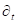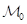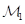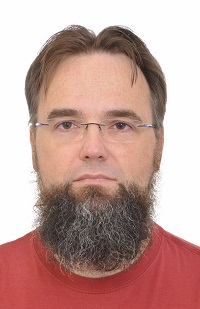Variational Methods for Evolutionary Systems
Speaker
Prof. Sebastian Franz
Technical University of Dresden, Germany
Abstract

Many linear (or linearised) problems of mathematical physics can be written as a first order system of the formwhereis the time-derivative,andare selfadjoint bounded operators andis a skew-selfadjoint, possibly unbounded operator that contains spatial derivatives like div, grad and curl.
In  a solution theory for above systems was developed and extended later to larger classes of problems. Classical models like wave-, heat-, reaction-diffusion-, acoustics, elasticity or Maxwell's equations all fall into this class, but also models that change their type within the domain.
We will consider in this talk variational discretisation methods for this class of problems, more precisely discontinuous  and continuous  FEM for time and space, and present a-priori convergence results.
An application of the theory will be within homogenisation of layered media, where hyperbolic and parabolic type problems are changing rapidly within one model, see e.g. [4,5].